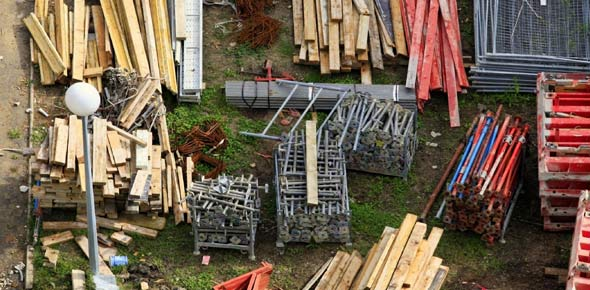# Materials 3

100 Questions | Total Attempts: 227Settings.

Related Topics
• 1.
After final rolling, the degree of compaction should be checked. The compated pavement shall have a density.
• A.

Equal to, or greater than 95% of the laboratory compacted density

• B.

Equal to, or greater than 97% of the laboratory compacted density

• C.

Equal to, or greater than 96% of the laboratory compacted density

• D.

Equal to, or greater than 94% of the laboratory compacted density

• 2.
The control of the temperature during mixing and compaction is of great significance.
• A.

The strength of the resulting pavement

• B.

The amount of the asphalt cement content

• C.

The amount of aggregate content

• D.

Proper determination of the method of rolling

• 3.
Calibration of universal testing machine shall be done.
• A.

Once in a year

• B.

Every 6 months

• C.

Every 4 months

• D.

Once a month

• 4.
Minimum size of class B for stone masonry
• A.

300mm

• B.

400mm

• C.

800mm

• D.

500mm

• 5.
Maximum slump for concrete class A
• A.

2"-5"

• B.

2"-4"

• C.

1"-2"

• D.

4"-9"

• 6.
Maximum water-cement ratio for concrete class A
• A.

0.46

• B.

0.53

• C.

0.55

• D.

0.58

• 7.
In a certain soil the LL=30 and PL=45. The plasticity index
• A.

15

• B.

-15

• C.

75

• D.

Non plastic

• 8.
In immersion/ compression test the ratio of wet to dry density of the mix
• A.

Stability

• B.

Index retained strength

• C.

Bulk specific gravity

• D.

Percent compaction

• 9.
Minimum required percent compaction for subbase course?
• A.

98%

• B.

95%

• C.

100%

• D.

96%

• 10.
In determining the grade of steel use
• A.

Tensile strength

• B.

Yield strength

• C.

Shear strength

• D.

Bond

• 11.
The concrete admixtures used when the normal setting time is shortened by the higher temperature, preventing the formation of cold joint, reduce the incidence of thermal cracking and to maintain concrete workability during large pours or long ready-mix hauls.
• A.

Accelerators

• B.

Retarders

• C.

Superplasticizers

• D.

• 12.
Angular coarse aggregate use for concrete mix will provide more.
• A.

Water content

• B.

Cement and water

• C.

Cement and fine aggregates

• D.

All of the above

• 13.
Required no. of representative core taken from each member of area in-place that is considered deficit
• A.

3

• B.

2

• C.

1

• D.

4

• 14.
Thickness determination of concrete cores shall be done at
• A.

4 measurements

• B.

9 measurements

• C.

6 measurements

• D.

8 measurements

• 15.
No. of set of concrete cylinder sample taken for every 75 cu.m of concrete
• A.

1 set

• B.

2 set

• C.

Any of a and b

• D.

3 set

• 16.
What will you recommend?
• A.

Reject

• B.

Recoring

• C.

Remove and replace

• D.

All of the above

• 17.
Most eefective factor for strength of concrete
• A.

Water-cement ratio

• B.

Water, cement and fine aggregates

• C.

Cement plus water

• D.

Any of the above

• 18.
Asphaltic materials use for treating an existing bituminous materials or cement concrete surface with bituminous materials
• A.

Prime coat

• B.

Tack coat

• C.

Seal coat

• D.

Any of the above

• 19.
Number of hours of concrete cylinder sample to be submitted to the laboratory before testing
• A.

24 hrs

• B.

48 hrs

• C.

12 hrs

• D.

72 hrs

• 20.
Number of hours of immersion/compression test that the sample place in water
• A.

4 hrs

• B.

6 hrs

• C.

7 hrs

• D.

8 hrs

• 21.
Required weight of sample for testing for coarse aggregates use for concreting
• A.

40 kg

• B.

50 kg

• C.

70 kg

• D.

80 kg

• 22.
Required weight of sample for testing for fine aggregates use for concreting
• A.

70 kg

• B.

50 kg

• C.

30 kg

• D.

40 kg

• 23.
The design thickness for PCCP is 200mm. The average thickness determination was taken and the result is 170mm. If you are a government materials engineer, what percent of contract price adjustment will you recommend?
• A.

70%

• B.

No payment

• C.

80%

• D.

85%

• 24.
The deficiency in concrete specimen was found out to be 19.5 percent. What is the adjust percent of contract price allowed.
• A.

80%

• B.

60%

• C.

70%

• D.

50%

• 25.
If there is a strength deficiency in the concrete specimens and it is not feasible or not advisable to obtain cores from the structure due to structural considerations, as a material engineer, what will you recommend?
• A.

Send letter to your superior for his decision

• B.

Recommend immediately adjusted price of payment with respect to the deficiency incurred

• C.

Both a and b

• D.

Tolerate strength deficiency